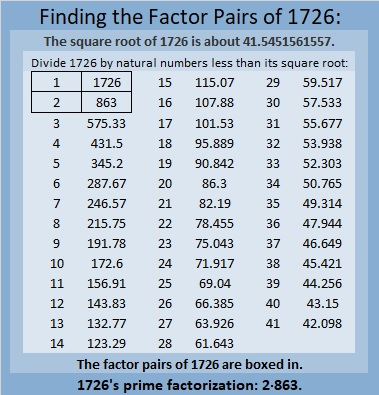# 1726 Find the Factors 1-12 AND 13-24

Contents

### Today’s Puzzle:

Here’s a puzzle I made to start off 2023. Either all the numbers from 1 to 12 will go in the top row OR they will all go in the first column. All the numbers from 13 to 24 will go in the remaining area. The possible factors are written on the side of the puzzle. Can you find all the factors? There is only one solution.### Factors of 1726:

• 1726 is a composite number.
• Prime factorization: 1726 = 2 × 863.
• 1726 has no exponents greater than 1 in its prime factorization, so √1726 cannot be simplified.
• The exponents in the prime factorization are 1 and 1. Adding one to each exponent and multiplying we get (1 + 1)(1 + 1) = 2 × 2 = 4. Therefore 1726 has exactly 4 factors.
• The factors of 1726 are outlined with their factor pair partners in the graphic below.### More About the Number 1726:

1726 is the sum of four consecutive numbers from 430 to 433.

This site uses Akismet to reduce spam. Learn how your comment data is processed.